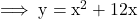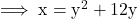## Identify the errors made in finding the inverse of y = x2 + 12x x= y2 + 12x y2 = x -12 y2 = -11x y= V-11x, for

Question

Identify the errors made in finding the inverse of
y = x2 + 12x
x= y2 + 12x
y2 = x -12
y2 = -11x
y= V-11x, for x 20
Describe the three errors

in progress 0
6 months 2021-07-21T20:12:28+00:00 1 Answers 16 views 0

x = y² + 12x

y² = x – 12

y² = -11x.

Step-by-step explanation:

We need to find the inverse of the given function , which is ,Step 1 : Interchange x and y :But according to the steps given in the Question , in very first step in 12x , x is not replaced by y . After which , the steps go wrong in the question .

The 3 errors :

• x = y² + 12x
• y² = x – 12
• y² = -11x.